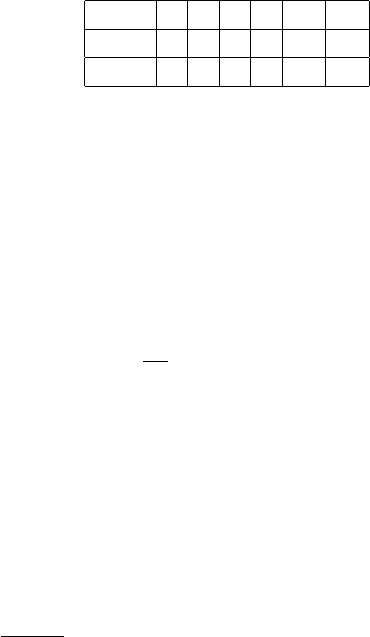Study Guides (390,000)
US (220,000)
UC-Irvine (3,000)
MATH (600)
MATH 2B (100)
All (60)
Final

# MATH 2B Final: MATH 2B Final Exam 2Exam

Department
Mathematics
Course Code
MATH 2B
Professor
All
Study Guide
Final

This preview shows pages 1-2. to view the full 7 pages of the document.MATH 2B: SAMPLE FINAL #2
This exam consists of 13 questions and 100 total points.
Read the directions for each problem carefully and answer all parts of each problem.
Please show all work needed to arrive at your solutions (unless instructed otherwise). Label
graphs and deﬁne any notation used. Cross out incorrect scratch-work.
No calculators or other forms of assistance are allowed. Do not check your cell phones
during the exam.
1. (8 points) Consider continuous functions fand f(where fdenotes the derivative of f) with
values given by the following table:
x0 1 2 3 4 5
f(x) 3 4 6 9 13 18
f(x) 1 2 4 6 7 5
a. Find R4
0f(x)dx
b. Estimate R4
1f(x)dx Using a left-hand Riemann sum with 3 equal subintervals.
c. Evaluate the following derivative at the point x= 3
d
dx Zx
2
f(t)dt
d. Suppose f(x)gives the height of a rocket, measured in yards, xminutes after its launch.
What are the units of R4
0f(x)dx and what does this quantity represent.
2. (7 points) Evaluate Zx
1 + x4dx

Only pages 1-2 are available for preview. Some parts have been intentionally blurred.3. (7 points) Evaluate Zx2
e2xdx
4. (7 points) Evaluate Rsin3(4t)dt